Reach Us+44-1522-440391
Locally Compact Homogeneous Spaces with Inner Metric | OMICS International
Journal of Generalized Lie Theory and Applications
All submissions of the EM system will be redirected to Online Manuscript Submission System. Authors are requested to submit articles directly to Online Manuscript Submission System of respective journal.

# Locally Compact Homogeneous Spaces with Inner Metric

Berestovskii VN*

Sobolev Institute of Mathematics SB RAS, Acad. Koptyug Avenue, 4 630090, Novosibirsk, Russia

Corresponding Author:
Berestovskii VN
Sobolev Institute of Mathematics
SB RAS, Acad. Koptyug Avenue
4630090, Novosibirsk, Russia
Tel: 865-974-5547
E-mail: [email protected]

Received date: January 05, 2015 Accepted date: June 29, 2015 Published date: July 07, 2015

Citation: Berestovskii VN (2015) Locally Compact Homogeneous Spaces with Inner Metric. J Generalized Lie Theory Appl 9:223. doi:10.4172/1736-4337.1000223

Copyright: © 2015 Berestovskii VN. This is an open-access article distributed under the terms of the Creative Commons Attribution License, which permits unrestricted use, distribution, and reproduction in any medium, provided the original author and source are credited.

Visit for more related articles at Journal of Generalized Lie Theory and Applications

#### Abstract

The author reviews his results on locally compact homogeneous spaces with inner metric, in particular, homogeneous manifolds with inner metric. The latter are isometric to homogeneous (sub-) Finslerian manifolds; under some additional conditions they are isometric to homogeneous (sub)-Riemannian manifolds. The class Ω of all locally compact homogeneous spaces with inner metric is supplied with some metric dBGH such that 1) (Ω, dBGH) is a complete metric space; 2) a sequences in (Ω, dBGH) is converging if and only if it is converging in Gromov-Hausdor sense; 3) the subclasses M of homogeneous manifolds with inner metric and L G of connected Lie groups with leftinvariant Finslerian metric are everywhere dense in (Ω, dBGH): It is given a metric characterization of Carnot groups with left-invariant sub-Finslerian metric. At the end are described homogeneous manifolds such that any invariant inner metric on any of them is Finslerian.

#### Keywords

Carnot group; Cohn-Vossen theorem; Gromov-Haudor limit; Homogeneous isotropy irreducible space; Homogeneous manifold with inner metric; Homogeneous space with integrable invariant distributions; Homogeneous (sub-)Finslerian manifold; Homogeneous (sub-)Riemannian manifold; Lie algebra; Lie group; Locally compact homogeneous geodesic space; Non-holonomic metric geometry; Rashevsky-Chow theorem; Shortest arc; Submetry; Symmetric space; Tangent cone

#### Introduction

One can observe in last decades an intensive development of nonholonomic metric geometry and its applications to geometric group theory, analysis, CR-manifolds, the theory of hypo-elliptic differential equations, non-holonomic mechanics, mathematical physics, thermodynamics, neurophysiology of vision etc. Montgomery's book  gives a well written track of this. A natural context for (sub-) Finslerian, in particular, (sub-)Riemannian geometry is geometric control theory [2,3].

Homogeneous Riemannian and Finslerian manifolds and their non-holonomic generalizations, homogeneous sub-Riemannian and sub-Finsleian manifolds, are especially important as models, because in some cases it is possible to find exactly geodesics, shortest arcs, conjugate and cut locus, and even distances for them.

A simple geometric axiomatic for homogeneous (sub-)Finslerian, in particular, (sub-)Riemannian, manifolds in general context of locally compact homogeneous spaces with inner metric, have been announced in paper . Later appeared proofs of this announcement [5-7], other interpretations, my later results and their survey , corrections to proofs of some results cited . Also last years some colleagues exhibited an interest in my old results . I am very obliged to professor Alekseevsky for useful discussions on this matter. Hopefully, all this serves as enough motivation to present a modified, renewed, relatively short (with omission of well-known definitions), version of some statements  and .

#### Locally Compact Homogeneous Spaces with Inner Metric

Let us remind main definitions. A path in a topological space X is a continuous map of some closed bounded interval of the real line to the space X. A metric space is called the space with inner metric, if the distance between any two its points is equal to the infimum of the length of paths joining these points. A metric space is homogeneous if its isometry group acts transitively, i.e., for any two points in the space there is an isometry (motion) of the space moving one of these points to the other.

The Cohn-Vossen theorem  states that every locally compact complete space (M, ρ) with inner metric is finitely compact, i.e., any closed bounded subset in (M, ρ) (in particular, any closed ball B(x, r) of radius r with the center at x) is compact; moreover, the space (M, ρ) is geodesic. The last statement means that any two points of the space can be joined by a segment or shortest arc, i.e., a curve (path) of length which is equal to the distance between these points.

Further we suppose that (M, ρ) is an arbitrary locally compact homogeneous space with inner metric, and G=I(M) is its motion group with compact-open topology with respect to its action on (M, ρ),G0 is a connected component of the unit in the group G. In view of homogeneity and the local compactness, the space (M, ρ) is metrically complete, the Cohn-Vossen theorem holds, and so we can use a shorter term “locally compact homogeneous geodesic space”.

The Busemann metric ,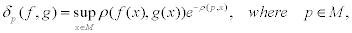is introduced on the group G.

The following results are proved in . The metric δp depends on the choice of the point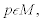, but it is bi-Lipschitz equivalent to the metric δp for any point q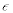M, and thus, independently on the point pM, defines a topology τ, which coincides with the compact-open topology on G with respect to its action on (M, ρ). Let us remind that the subbasis of the compact-open topology consists of sets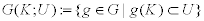, where K is a compact and U is an open subset in M. The metric δp is invariant under the left translations by elements of the group G and under the right translations by elements of its compact subgroup H, the stabilizer of the groups G at a point p; for natural identification of G/H with M; defined by formula σ(gH)=g(p), the quotient metric Δp on G/H, induced by the metric δp, is equivalent to the metric ρ, the metric space (G, δp) is locally compact, complete, and separable. The pair (G,τ) is a topological group acting continuously and properly on the left on (M, ρ) by isometries. The subgroup G0 is transitive on M.

The following interesting problem is still open.

Problem 1. Is it true, that in the general case, the connected group G0 or another transitive on M closed connected subgroup of the group G is locally connected or, which is equivalent, locally arcwise connected? .

Observe in relation with this, that the paper  gives a very short proof of a new (at that time) result, that is a global form of a theorem on the local representation of a group as a direct product coming from the Iwasawa-Gleason-Yamabe theory [13-15] for locally compact groups.

Theorem 1. Let G be a connected locally compact (Hausdorff) topological group. Then there exists a compact subgroup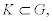a connected, simply connected Lie group L, and a surjective local isomorphism π: K x L → G Furthermore, if G is locally connected, then K is connected and locally connected, and π is a covering epimorphism.

The following characterization of locally compact homogeneous geodesic spaces as homogeneous spaces of topological groups  holds.

Theorem 2. Every locally compact homogeneous geodesic space is isometric to some locally compact locally connected quotient space G/H of a connected locally compact topological group G with the first countability axiom, by a compact subgroup H, endowed with a G-invariant geodesic metric.

Conversely, every locally connected, locally compact homogeneous quotient space G/H of a connected locally compact topological group G with the first countability axiom by a compact subgroup H admits a G-invariant geodesic metric ρ.

Corollary 1. A locally compact topological group (G, τ) admits some left-invariant geodesic metric if and only if (G, τ) is connected, locally connected, and satisfies the first countability axiom.

Theorem 3. Any neighbourhood U of the unit e in a connected locally compact topological group G contains closed (even compact) normal subgroups N=NU with the quotient group G/N, which is a (connected) Lie group.

Lemma 1. If N1 and N2 are normal subgroups of a locally compact topological group G such that G/N1 and G/N 2 are Lie groups, then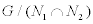is also a Lie group .

We need the following definition in order to formulate other structural results on locally compact homogeneous geodesic spaces.

Definition 1. A map of metric spaces f : M → N is said to be submetry, if for any point x ∈ M, and any number r > 0, we have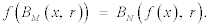Here B denotes the closed ball of corresponding radius in the corresponding space .

Theorem 4. Any Riemannian submersion of complete smooth Riemannian manifolds is a submetry. Conversely, a submetry of smooth Riemannian manifolds is the Riemannian submersion of class C1, 1 

On the ground of theorems 2, 3, lemma 1, and definition 1, we prove the following

Theorem 5. A metric space (M, ρ) is a locally compact homogeneous geodesic space, if and only if, it can be represented as the inverse metric limit of some sequence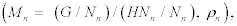where Nn is non-increasing sequence of compact normal subgroups of G such that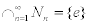of homogeneous geodesic manifolds bound by the proper (the preimage of a compact set is compact) submetries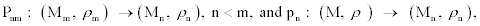where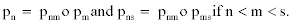[4,6].

This means that (non-decreasing) functions ρn o (pn x pn) uniformly converge to the metric ρ. Under this condition pnm ∈ C, and one can assume that the fibers of these submetries are connected.

In some sense, Theorem 5 reduces the study of locally compact homogeneous geodesic spaces to the case of homogeneous geodesic manifolds.

Let us recall, that the Hausdorff distance dH (A, B) between two bounded subsets of an arbitrary metric space M is the infimum of positive numbers r, such that A is contained in the r-neighbourhood of the set B, and B is contained in the r-neighbourhood of the set A. The pair (K(M), dH ) is a metric space where K(M) is the family of all closed bounded subsets of the metric space M. It is complete if the space M is complete .

Definition 2. The Gromov-Hausdorff distance dGH (A, B) between compact metric spaces is defined as the infimum of all distances dH(f(A), g(B)) for all metric spaces M, and for all isometric embeddings f : A → M, and g : B → M. By definition, a sequence ((Xn, xn), ρn) of finitely compact complete spaces with metrics ρn and chosen points xn Gromov- Hausdorff-converges to a similar space ((X, x), ρ), if for any number r > 0, the distance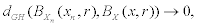as n → +∞.

Definition 3. The distance dBGH between finitely compact metric spaces with chosen points (X, x) and (Y, y) is equal by definition to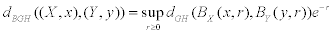As a consequence of S. E. Cohn-Vossen theorem, cited above, this definition is applicable to locally compact complete spaces with inner metric, in particular, to locally compact homogeneous spaces with inner metric. It is clear that in the latter case the distance dBGH does not depend on the choice of points x ∈ X and y ∈ Y .

Let Σ and Θ be respectively the classes of all finitely compact metric spaces and locally compact complete inner metric spaces with chosen points, and let Ω be a class of all locally compact homogeneous spaces with inner metric.

Theorem 6. The pair (Σ , dBGH ) is a complete metric space. The convergence of sequences in this metric space is equivalent to the Gromov-Hausdorff convergence. So Θ and Ω are closed subspaces of (Σ, dBGH). Moreover, the subclass M of homogeneous manifolds with inner metric is everywhere dense in (Ω, dBGH).

#### Homogeneous Manifolds with Inner Metric

Theorem 7. The following statements for locally compact homogeneous space with inner metric (M, ρ ) are equivalent:

(1) M is a (connected) topological manifold;

(2) M has finite topological dimension;

(3) M is locally contractible;

(4) (M, ρ) is isometric to (G/H, d); where G is a connected Lie group, H is a compact Lie subgroup of G, and d is some inner metric on G/H; invariant relative to the canonical left action of G on G/H.

Let us give some explanations. Evidently, (1) implies (2) and (3); (4) implies other statements. Now (2), theorems 2 and 5 imply that M=G/H; where G is a locally compact, connected topological group such that some neighborhood U of e contains no nontrivial normal subgroup, and H is a compact subgroup of G. Then theorem 3 implies that G is a connected Lie group, H is a compact Lie subgroup of G; which proves (4).

The statement (3) together with theorem 2 would imply the statement (4) by Szenthe's claim in : let a σ-compact locally compact group G, with a compact quotient G/G0; acts continuously (and properly) as a transitive and faithful transformation group on a locally contractible space X. Then X is a manifold and G is a Lie group.

However, it was discovered in 2011 by Antonyan , that Szenthe's proof of this claim contains a serious gap. Independently Szenthe's claim was proved by Antonyan and Dobrowolski , by Homann and Kramer , see also the book by Hoffmann and Morris .

Theorem 7 gives topological characterization of homogeneous manifolds with inner metric. Now we shall describe their metric structure.

Let M=G/H be the quotient manifold of a connected Lie group G by its compact Lie subgroup H; g=Ge, h=He be Lie algebras of Lie groups G, H: Let us set the following objects:

(a1) Le is Ad(H)-invariant vector subspace of g such that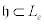and g is the least Lie subalgebra of g which contains Le;

(a2) DH=dp(e)(L0); where p: G → G/H is the canonical projection and dp is its differential;

(a3) FH is a norm on DH which is invariant relative to the (linear) isotropy group of G/H at H ∈ G/H;

(a4) D is G-invariant distribution on G/H such that D(H)=DH ;

(a5) F is G-invariant norm on the distribution D such that F (H)=FH.

Theorem 8. Let M=G/ H be the quotient space of a connected Lie group G by its compact Lie subgroup H, (D, F) is a pair with conditions (a1) (a5) [4,5,7].

Then the formula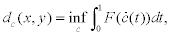where c=c(t), 0 ≤ t ≤ 1, are arbitrary piecewise smooth paths in G/H, tangent to distribution D and joining points x and y from G/H; defines some G-invariant geodesic metric dc on G/H (compatible with the standard topology on G/H).

Remark 1. Conditions (a1), (a2), and (a4) imply that the distribution D from theorem 8 is completely nonholonomic . Therefore any two points x and y from G/H can be joined by some piecewise smooth path by Rashevsky-Chow theorem [24,25], so dc(x, y) is finite. If D=TM (respectively, D ≠ TM) then dc is said to be (sub-)Finslerian and (sub-) Riemannian if additionally FH is an Euclidean norm on DH : Note that a norm || · ||on a vector space V is Euclidean if and only if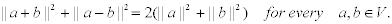Theorem 9. Every G-invariant inner metric on a homogeneous manifold G/H of connected Lie group G by its compact subgroup H is sub-Finslerian or Finslerian. In addition, the Lie group G admits a leftinvariant sub-Finslerian or Finslerian (sub-Riemannian or Riemannian if is sub-Riemannian or Riemannian) metric ρ0 such that the canonical projection p: (G, ρ0) → (G/H, ρ) is submetry [5,7].

Using this theorem, it is not difficult to prove the following addition to theorem

Theorem 10. The class L G of connected Lie groups with leftinvariant Finslerian metric is everywhere dense in ( Ω, dBGH ) .

The last statement of theorem 9 implies that the search of geodesics and shortest arcs of invariant (sub-) Finslerian or (sub-) Riemannian metric on homogeneous manifolds reduces in many respects to the case of Lie groups with left-invariant (sub-) Finslerian or (sub-) Riemannian metric.

Any shortest arc, parametrized by the arc-length, on (G, ρ0) from theorem 9 is a solution of a time-optimal problem; so it necessarily satisfies the Pontryagin maximum principle (PMP) [4,26]. Unfortunately, this principle is useful only for so-called normal shortest arcs and geodesics, when a maximum, supplied for them by PMP, is positive. Every normal geodesic on (G, ρ0) is smooth if ρ0 is sub-Riemannian metric; moreover, if any geodesic on (G, ρ0) is normal (which is always true if ρ0 is Riemannian) then any geodesic on (G/H, ρ) is smooth.

Let us note that using PMP, the author found in paper  all geodesics and shortest arcs of arbitrary left-invariant sub-Finslerian metric on three-dimensional Heisenberg group.

#### Tangent Cones and Carnot Groups

Definition 4. A bijection of metric space (M, ρ) onto itself is called a (metric) a-similarity, if ρ (f(x), f(y))=a ρ(x, y) for all points x, y ∈ M, where a∈<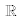, a > 0. The a-similarity is called nontrivial, if a ≠ 1.

Theorem 11. A locally compact homogeneous space with an inner metric (M, ρ) admits nontrivial metric similarities if and only if (M, ρ) is isometric to a finite-dimensional normed vector space or to a Carnot group, i.e. connected, simply connected, (noncommutative) nilpotent stratified Lie group C with the Lie algebra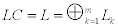(of nilpotentness depth m>1), which is a direct sum of vector subspaces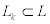under the conditions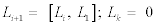if k>m; with leftinvariant sub-Finslerian metric dcc, defined by a left-invariant norm Fc on the left-invariant distribution Δ, where Δ (e)=L1. Moreover, (M, ρ) admits a-similarities for all positive a ∈<[28,29].

Theorem 12. If (M, ρ) is a homogeneous manifold with inner metric then at any point x ∈ (M, ρ) there exists the tangent cone τxM to the manifold (M, ρ) (in the Gromov's sense) as the Gromov-Hausdorff limit of spaces ((M, x) αρ ) when α →+ ∞. Let suppose that (M, ρ) is (M=G/H, dc) as in theorem 8. If dc is Finslerian metric, defined by the norm F0 on DH=THM, then τxM is isometric to the normed vector space (TH M, F0); otherwise τxM is isometric to a Carnot group (C, dcc); where normed vector spaces (L1, Fc) and (DH, F0) are isometric [30,31].

Let us note that it follows from theorem 6 and the first statement of theorem 12 that τxM is a locally compact homogeneous space with inner metric which has a-similarities for every positive number a. Now other statements of theorem 12 could be deduced from theorem 11.

#### Homogeneous Finsler Manifolds

Theorem 13. A metric space (M, ρ) is isometric to a homogeneous Finslerian manifold if and only if (M, ρ) is locally compact homogeneous space with inner metric of finite topological dimension which is equal to its Hausdorff dimension .

Proof. The necessity of these conditions is well-known.

Sufficiency. By theorems 7, 9, every locally compact homogeneous space with inner metric (M, ρ) of finite topological dimension is a (sub-)Finslerian homogeneous manifold, defined by conditions from theorem 8.

If FH from theorem 8 is not Euclidean, then we can find an Euclidean norm F1H on DH , invariant relative to the (linear) isotropy group of G/H at H ∈ G/H. Then there is a constant c > 1 such that (1/c) F1H ≤ FH ≤ cF1H.Now let ρ1=d1c be G-invariant (sub-)Riemannian metric on G/H defined by formula (2), where F is G-invariant norm on D such that F (H)=F1H : Then it is easy to see that (1/c) ρ1 ≤ ρ ≤ cρ1 and therefore (M, ρ)and (M, ρ1) have equal Hausdorff dimensions.

The space (M, ρ) is Finslerian if and only if (M, ρ1) is Riemannian. To finish proof it is enough to apply for (M, ρ1) theorems 12, 11, and known facts that so-called equiregular connected smooth sub- Riemannian manifold M and any its tangent cone τxM have equal Hausdorff dimensions, while the Hausdorff dimension of the Carnot group (C, dcc) from theorem 11 is equal to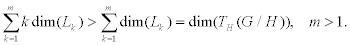Theorem 14. Every Lie group with bi-invariant (i.e. with leftand right-invariant) inner metric is the Lie group with bi-invariant Finslerian metric [4,5].

#### Homogeneous (sub-)Riemannian Manifolds

Theorem 15. A metric space (M, ρ) is isometric to a homogeneous Riemannian manifold if and only if (M, ρ) is a locally compact homogeneous space with inner metric of finite topological dimension which has the curvature ≥ K in A. D. Alek sandrov's sense for some K ∈<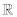.

Notice that there are different equivalent definitions of Aleksandrov spaces of curvature ≥ K [32,33]. The following definition belongs to the author.

Definition 5. A space M with an inner metric and with the local ex-istence of shortest arcs is called the Aleksandrov space of curvature ≥ K if locally any quadruple of points in M is isometric to some quadruple of points in a simply connected complete Riemannian 3-manifold of some constant sectional curvature k ≥ K, where the number k depends on considered quadruple of points [12,34].

Remark 2. There are infinite dimensional compact homogeneous spaces with inner metric of Aleksandrov curvature ≥ 0 . A smooth Riemannian manifold has Aleksandrov curvature ≥ K if and only if its sectional curvatures ≥ K. The definition 5 is local, but every quadruple of points in geodesic Aleksandrov space of curvature ≥ K in a sense of this definition satisfies conditions from definition 5 . Some other conditions, in terms of orbits of 1-parameter subgroups of isometries, characterizing homogeneous Finsler and Riemannian manifolds, are given in papers [4,7].

I don't know simple metric conditions, characterizing homogeneous sub-Riemannian manifolds, aside as the Gromov-Hausdorff limits of homogeneous Riemannian manifolds, when limits have different finite topological and Hausdorff dimensions.

It is interesting that there is a probabilistic approach to solve this problem, at least in the case of left-invariant inner metrics on Lie groups.

Theorem 16. Left-invariant (sub-)Riemannian metrics on a connected Lie group are in 1-1 correspondence with symmetric Gaussian 1-parameter convolution semigroups of {e}-continuous, absolutely continuous with respect to left-invariant Haar measure, probability measures on it [8,35].

Omitting details, we reference to exact definitions and theorem 6.3.8 in book  which characterizes generating infinitesimal (hypo-) elliptic operators of such semi-groups. Notice that there is no mention to left-invariant (sub-)Riemannian metrics on Lie groups .

Problem 2. It would be desirable to get a generalization of theorem 6.3.8 in  to the case of homogeneous manifolds G/H and use it for (sub-)Riemannian geometry.

#### Homogeneous Manifolds with Integrable Invariant Distributions

In this section we consider very natural problem: describe connected homogeneous manifolds such that every invariant inner metric on any of them is Finslerian.

Theorem 17. Every G-invariant inner (geodesic) metric on the homogeneous space G/H of a connected Lie group G with a compact stabilizer H ⊂ G is Finslerian if and only if [4,7]

(A) Every G-invariant distribution on G/H is integrable.

This is equivalent to the condition

(B) Every Ad(H)-invariant vector subspace c in g containing h is a Lie algebra.

If H is connected, in particular, if G/H is simply connected, then the Ad(H)- invariance of the space c is equivalent to the inclusion [h, c] ⊂ c.

Definition 6. The homogeneous manifold G/H of a connected Lie group G with a compact stabilizer H is called homogeneous manifold with integrable invariant distributions, shortly, HMIID, if it satisfies any of the equivalent conditions (A) or (B) from theorem 17.

Theorem 18. The following conditions for a connected Lie group G with the Lie algebra g are equivalent:

1) Every left-invariant inner metric on the Lie group G is Finslerian;

2) Every vector subspace of the Lie algebra g is a Lie subalgebra in g;

3) g is one-dimensional or any two-dimensional vector subspace in g is a Lie subalgebra of g;

4) For any two elements X, Y in g, the bracket [X, Y ] is a linear combination of elements X and Y [4,7].

Theorem 19. If a Lie algebra g satisfies condition 4) from theorem 18 then

1) there exists a linear map l : g→ R such that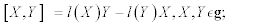2) the kernel of the linear map l is the maximal commutative ideal in g;

3) l=0 if and only if g is commutative Lie algebra;

4) if l ≠ 0 then, up to an isomorphism, the Lie algebra g has the form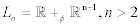i.e. semidirect sum, prescribed by homomorphism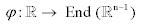Such that Φ(1)=En-1 is the unit matrix.

Theorem 20. Let Gn be n-dimensional Lie group G with the Lie algebra g, satisfying condition 4) from theorem 18 . Then

1) Gn is commutative or

2) Gn is isomorphic to the group of real (n n) block matrices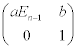where En-1 is the unit (n-1) x (n-1)-matrix, a is any positive number, b is any (n-1)-vector-column, and 0 is the zero (n-1)-vector-row.

Theorem 21. Noncommutative Lie group G has the Lie algebra g satisfying condition 4) from theorem 18 if and only if any left-invariant Riemannian metric on G has constant negative curvature .

Theorem 22. Let M be a connected Riemannian symmetric space, G be the maximal connected Lie group of isometries for M with the stabilizer H⊂ G at a point x∈ M: Then any G-invariant inner metric on G/H is Finslerian [4,7].

Theorem 23. Let assume that M=G/H (where G is a connected Lie group and H is its compact subgroup) be isotropy irreducible homogeneous spaces, i.e. G/H has irreducible linear isotropy group. Then any G-invariant inner metric on G/H is Finslerian [4,7].

Theorem 24. For any (compact) simply connected effective homogeneous space G/H of a connected compact Lie group G with closed stabilizer H the following conditions are equivalent [37-39]:

1) all G-invariant distributions on G/H are integrable;

2) the homogeneous space G/H is isomorphic to a direct product of compact simply connected isotropy irreducible homogeneous spaces;

3) the space G/H has normal type by Berard-Bergery , i.e. any G-invariant Riemannian metric on G/H is normal homogeneous in M.Berger's sense;

4) all G-invariant Riemannian metrics on G/H have positive Ricci curvature;

5) all G-invariant Riemannian metrics on G/H have positive scalar curvature.

Simply connected irreducible (Riemannian) symmetric spaces G/H with connected Lie group G and compact subgroup H have been classiffed by Cartan . They are (automatically) strictly isotropy irreducible, i.e. H0 has irreducible isotropy representation; non-compact strictly isotropy irreducible homogeneous spaces are symmetric . Manturov  and Wolf  classified strictly isotropy irreducible homogeneous spaces; one needs to combine their results to get full classification .

The author used no classification when he proved his results stated in this paper. It follows from previous statements that there is a full classification of compact simply connected HMIID.

Gorbatsevich [8,44] studied general homogeneous spaces with connected stabilizer subgroup from the class HMIID in detail. He described corresponding transitive Lie groups and stabilizer subgroups in the case when the transitive group is semisimple or solvable, and partly, in the case of general transitive Lie groups.

#### References

Select your language of interest to view the total content in your interested language

### Article Usage

• Total views: 12267
• [From(publication date):
July-2015 - Aug 21, 2019]
• Breakdown by view type
• HTML page views : 8382
• PDF downloads : 3885

## Post your commentCan't read the image? click here to refresh
###### Peer Reviewed Journals

Make the best use of Scientific Research and information from our 700 + peer reviewed, Open Access Journals

International Conferences 2019-20

Meet Inspiring Speakers and Experts at our 3000+ Global Annual Meetings

Top